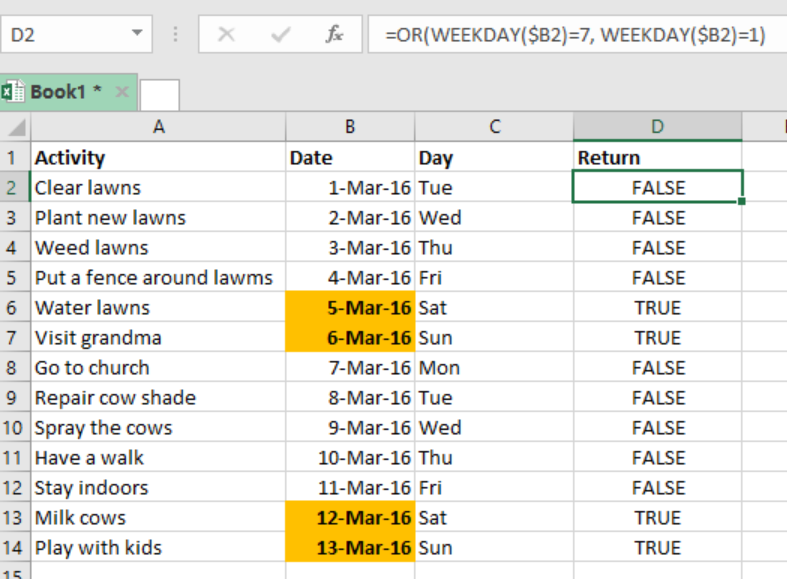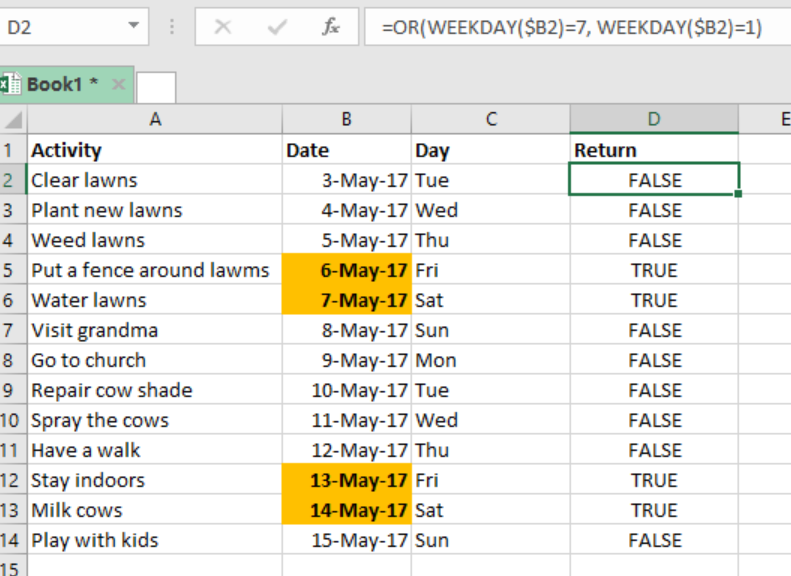Get instant live expert help with Excel or Google Sheets“My Excelchat expert helped me in less than 20 minutes, saving me what would have been 5 hours of work!”

#### Post your problem and you’ll get Expert help in seconds.

Your message must be at least 40 characters
Our professional Expert are available now. Your privacy is guaranteed.

# Highlight dates that are weekends

There are times when we want to highlight only those dates that are weekends in excel. To do this, we can use a formula that is based on WEEKDAY function. This post provides a guide on how to use the WEEKDAY function to highlight dates that are weekends.Figure 1. Final result

## General syntax of the formula

`=OR(WEEKDAY(A1)=7,WEEKDAY(a1)=1)`

## Understanding the formula

When we have specific activities set for the weekends, and we want to highlight them so that we can remember, we can utilize a formula that is based on the OR and WEEKDAY functions. The above formula will help us highlight weekends, i.e. Saturday and Sunday.

In our example above, we have the dates in column B, i.e. B2:B14. To get the dates that are weekends, we have use the formula below;

`=OR(WEEKDAY(B2)=7, WEEKDAY(B2)=1)`

## How the formula works

The formula utilizes the WEEKDAY function to check whether supplied dates are Saturdays and Sundays.

• The WEEKDAY function returns a number, 1-7 for each of the dates supplied.
• Usually, the function assigns Saturday a 7, while Sunday a 1.
• The OR function will then test a 1 or 7.
• If either of 1 or 7 is true among the dates, then our formula returns TRUE. If none is true, the formula returns a FALSE.

## How to highlight the weekend dates rows

For you to highlight the weekend dates, you will have to use conditional formatting rule to all the columns in the table, and lock the date column.

## ExampleFigure 2. Example

In this example, we want to highlight the weekends so that we can plan our activities for those days. To do this, we proceed as follows;

Step 1: Prepare the worksheet, including the activities, date for the activities, day and what to be returned by the formula.

Step 2: Apply conditional formatting to the date column (B), as we shall want the weekends to be highlighted in a different color.

Step 3: In cell D2, put the formula `=OR(WEEKDAY(\$B2)=7, WEEKDAY(\$B2)=1)`

Step 4: Press Enter to get either TRUE or FALSE.

Step 5: Copy down the formula to the other cells.

## Instant Connection to an Expert through our Excelchat Service

Most of the time, the problem you will need to solve will be more complex than a simple application of a formula or function. If you want to save hours of research and frustration, try our live Excelchat service! Our Excel Experts are available 24/7 to answer any Excel question you may have. We guarantee a connection within 30 seconds and a customized solution within 20 minutes.

### Did this post not answer your question? Get a solution from connecting with the expert.Another blog reader asked this question today on Excelchat:
Solution examplesUse the Vlookup Function to complete the "employee" column of table 2. Use "job Id" from table 2 as your lookup_value(s) and table 1 as your reference.
Solved by C. H. in 16 minsI have a list or people who are ranked in numbers from 3 to 6 I need to recognize the contents of each multiple cells and create an equivalent letter. for that value into another column. e.g. Cell E1 = 3 to show in new cell that row (H1) the letter "C" I have multiple rows with different values in column "E" Can this be done?
Solved by I. J. in 30 minsIf a cell in another sheet is populated I need a vlookup done. If the cell is not populated I need the cell to return blank.
Solved by T. D. in 60 minsI am trying to make a chart that turns a week range red if nothing is entered in the range. If something is entered then I would like it to turn green. Please Help
Solved by E. U. in 43 minsI need a check box to show/hide an answer of an if function
Solved by Z. U. in 23 mins## Subscribe to Excelchat.coAnother blog reader asked this question today on Excelchat: